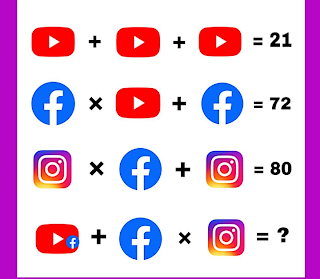0

This Social Media puzzle is making rounds online and many people failed it. Today I will solve it in detail and give you the correct answer.

## Tricky social media puzzle:

The trick in Many puzzles is interpretation.

Instagram × Facebook + Instagram = 80

Then,

See picture belowI will solve each equation and substitute it in the final equation to get the correct answer.

Equation one:  3 YouTube = 21

Implies YouTube = 7  simple right? The others are a bit tricky, pay attention!

Equation two

Facebook(7 + 1 ) = 72

Equation three

Instagram× 9 + Instagram = 80

Instagram ( 9+1)= 80

Instagram = 80÷( 9+1)

Instagram = 80÷10

Instagram = 8.

Instagram = 8.

I will substitute in the final equation and simplify  to get the final answer to the tricky social media math puzzle.

Final equation

(7+9) + 9 ×8=?

16 + 9× 8=?

88=?

Therefore the correct answer to the social media Math puzzle is 88.